## Tuesday, 8 March 2022

by Giovanni Carrera, 3/03/2022

ESP32 has two 12-bit SAR (Successive Approximation Register) ADCs which are able to convert up to 18 analog inputs. The maximum value to convert is equal to the reference value Vref, for the ESP32 it is about 1 V, but it can vary between 950 and 1100 mV. It is possible to use voltages higher than Vref by attenuating the input. The ESP32 has four possible attenuation options:

 Attenuation input voltage range ADC_ATTEN_DB_0 100 mV ~ 950 mV ADC_ATTEN_DB_2_5 100 mV ~ 1250 mV ADC_ATTEN_DB_6 150 mV ~ 1750 mV ADC_ATTEN_DB_11 150 mV ~ 2450 mV

In my case, the default attenuation is 0 dB, with an input range of about 68 to 995 mV. For better accuracy it is recommended not to use attenuation, ie 0 dB, and to employ the pins of ADC1, I used GPIO34.

Figure 1 shows a fairly serious problem on low input levels: below 68 mV the converter output gives zero, creating a dead zone and strong non-linearity.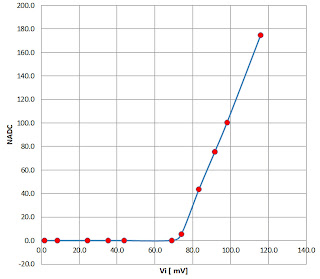figure 1

So I tried to think of a circuit able to accept an input down to zero volts and to output a signal that can be converted without the drawbacks described above. If, for example, we want to have the following conditions:

Vin = 0 mV → Vout = 100 mV

Vin = 3000 mV → Vout = 1000 mV

The summer circuit, shown in figure 2, realizes these conditions very well.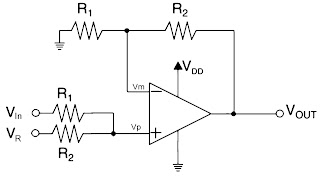Figure 2

Remembering that for an ideal op amp the inputs are at the same potential, so Vm = Vp , its output voltage is:

Vout = Vp(1+R2/R1)   (1)

Where Vp is the voltage on the non-inverting input of the operational amplifier:

Vp = Vin –(Vin -VR)*R1/(R2+R1)       (2)

In the case of VIN = 0,  we have:

Vp = VR*R1/(R2+R1)

Setting VR = 100 mV, R1 = 300 k and R2 = 100 kΩ, we obtain:

Vout = Vp*4/3

Vp = VR*3/4 = 75 mV

Therefore the output voltage is:

Vout = VR*3/4*4/3 = VR = 100 mV

In the case of VIN = 3000 mV,  from the expression 2 we have:

Vp = (VIN - VR) *R2/(R2+R1) + VR

And, substituting, we have:

Vp = 3000 –(2900)*3/4= 825 mV

So the output voltage is:

Vout = Vp*4/3 =1100

Setting R1 = 330 kand R2 = 100 kΩ, the conditions imposed are almost perfectly met:

Vin = 0 mV → Vout = 100 mV

Vin = 3000 mV → Vout = 1009 mV

Figure 3 shows the wiring diagram of my prototype. The operational amplifier U1A serves as a voltage follower, U1B as an adder, and U2 provides a stable reference voltage. In my prototype I used the values indicated in the diagram, but I suggest to use the value of 330 k for R2 and R3.

Rail-to-rail operational amplifiers, such as the one indicated, are particularly suitable for this application.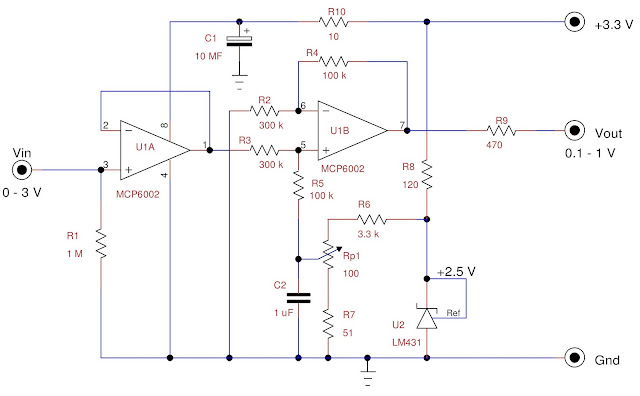Figure 3

Now you need to perform a calibration. First you have to write a program that reads the values of an analog pin, in my case IO34, and prints them. You can use the Arduino IDE or MicroPython, as in my case.

Put a jumper between the input and ground for Vin = 0, then slowly turn the trimmer Rp1 until you begin to see numbers other than zero.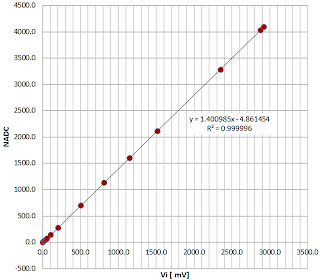Figure 4

We then connect the Vin input to a low-noise, stable voltage generator, checking the voltage with an accurate digital voltmeter. I take the various measurements and put them on an Excel-like spreadsheet to do a linear regression like the one in figure 4. As can be seen, the results were excellent, with an R2 very close to one. To have the millivolts output, you need to invert the axes of the regression and insert the following expression:

Obviously these values are valid in my case.

Components list

 component description component description R1 1 MW ± 1% metal film R10 10 W ± 5% R2, R3 330 kW ± 1% metal film Rp1 100 Ω multi-turn trimmer R4, R5 100 kW ± 1% metal film C1 10 µF,35V Aluminum electrolytic R6 3.3 kW ± 1% metal film C2 1 µF,25V ceramic AVX R7 51 W ± 1% metal film U1 MCP6002, dual rail-to-rail op amp R8 120 W ± 5% U2 TL431, shunt voltage reference R9 470 W ± 5%

Program

This program, written in MicroPython, acquires 100 samples at 500 Hz, makes the statistics and prints them. I took the average value while also observing the standard deviation of the measurements which must be minimal.

# Giovanni Carrera, 03/03/2022

from time import sleep,sleep_ms

import math

ndata = 100

arr = [0 for i in range(ndata)]

while True:

vmean = 0

vqmean = 0

vmax = 0

vmin = 5000

for x in range(0,ndata):

if arr[x] > vmax :

vmax = arr[x]

if arr[x] < vmin :

vmin = arr[x]

vmean += arr[x]

vqmean += arr[x]**2

sleep_ms(2)

vmean /= ndata # mean value

vqmean /= ndata # mean of quadratic values

k = vqmean - vmean**2

if k >= 0 :

StDev = math.sqrt(k)

else :

StDev = 0

print('Analog ch = IO34')

print('Max =' + str(vmax))

print('Min=' + str(vmin))

print('Mean=' + str('%.1f' %vmean))

print('Sdev=' + str('%.1f' %StDev))

print()

sleep(1)

Reference

1.       “AN682 Using Single Supply Operational Amplifiers in Embedded Systems”, Bonnie Baker, Microchip Technology Inc. , 2000

“ESP32 Technical Reference Manual, Version 3.0”, Espressif Systems

1.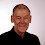This is an interesting article for me, however the code for calibration is written in MicroPython. I would appreciate very much an Arduino code for Arduino Ide. Would that be possible for you to publish?

1.This simple program print an ADC value every 0.5 s:
void setup(void) {
Serial.begin(115200);
}
void loop() {